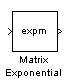# Matrix Exponential

Compute matrix exponential

• Library:
• DSP System Toolbox / Math Functions / Matrices and Linear Algebra / Matrix Operations

•## Description

The Matrix Exponential block computes the matrix exponential of a square matrix using a scaling and squaring algorithm with a Pade approximation.

## Ports

### Input

expand all

Specify the input data as a square matrix.

Data Types: `single` | `double`
Complex Number Support: Yes

### Output

expand all

Matrix exponential output of the input square matrix.

Data Types: `single` | `double`
Complex Number Support: Yes

## Block Characteristics

 Data Types `double` | `single` Direct Feedthrough `no` Multidimensional Signals `no` Variable-Size Signals `no` Zero-Crossing Detection `no`

## Extended Capabilities

### Blocks

Introduced before R2006a

## SupportGet trial now